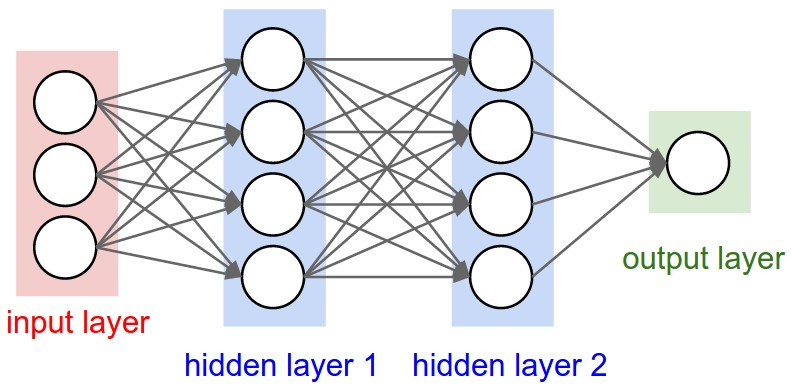2 views

For any Keras layer (Layer class), can someone explain how to understand the difference between input_shape, units, dim, etc.?

For example, the doc says units specify the output shape of a layer.

In the image of the neural net below hidden layer1 has 4 units. Does this directly translate to the units attribute of the Layer object? Or does units in Keras equal the shape of every weight in the hidden layer times the number of units?

In short, how does one understand/visualize the attributes of the model - in particular the layers - with the image below?by (108k points)

Units are the number of neurons contained in each layer. It's a property of each layer, and yes, it's related to the output shape. In your above image, except for the input layer, you have:

• Hidden layer 1: 4 units (4 neurons)

• Hidden layer 2: 4 units

• Last layer: 1 unit

Answering your question, yes it directly translates to the unit attribute of the layer object.

Shapes are consequences of the model's configuration. Shapes are tuples, representing the number of elements an array or tensor has in each dimension.

For example, a shape(30,4,10) means an array of a tensor with 3 dimensions, containing 30 elements in the first dimension, 4 in the second and 10 in the third, totaling 30*4*10 = 1200 elements or numbers.

Tensor is the element that flows between the layers. Tensors can be represented as matrices, with shapes. In Keras, the input is a tensor, not a layer. This tensor must have the same shape as your training data.

If you have 30 images of 50x50 pixels in RGB (3 channels i.e. red, green and blue), the shape of your input data is(30,50,50,3). Then your input layer tensor must have the shape which is mentioned in the above example.

Each layer requires the input with a particular number of dimensions:

1. Dense layer requires the input as (batch_size, input_size)

2. 1-dimensional layer requires the input as (batch_size, length_of_sequence, features)

3. 2-dimensional layer requires the input as follows:

• If using channel first: (batch_size, channels, image1, image2)

• If using channel last: (batch_size, image1, image2, channels)

You already know about the input shape (seeing your training data), you must define it to the model as it cannot learn itself about the input shape. All the other shapes are calculated automatically based on the units and particularities of each layer.

Units: It defines the output shape i.e. the shape of the tensor that is produced by the layer and that will be the input of the next layer. Dense layers have the output based on the units.

Now coming to the dim part.

Dim is the tuple of integers. If your input shape has only one dimension, then you don't have to give it as a tuple, you will have to give it as a scalar number. Now if your model has an input layer containing 3 elements, you can use any of these two syntaxes:

• input_shape=(3, )The comma is necessary when you have only one dimension

• input_dim=3

But when it comes to the tensor, dim is often considered as the dimensions like shape(20,39) has 2 dimensions.

Go through the Artificial Intelligence Course to get clear understanding of Keras input explanation.